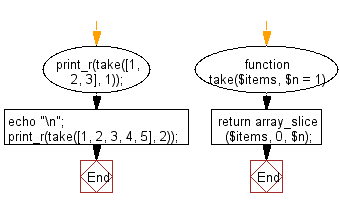﻿ PHP Exercise: Get an array with n elements removed from the beginning of a given array - w3resource

# PHP Exercises: Get an array with n elements removed from the beginning of a given array

## PHP: Exercise-91 with Solution

Write a PHP program to get an array with n elements removed from the beginning of a given array.

Sample Solution: -

PHP Code:

``````<?php
//Licence: https://bit.ly/2CFA5XY

function take(\$items, \$n = 1)
{
return array_slice(\$items, 0, \$n);
}
print_r(take([1, 2, 3], 1));
echo "\n";
print_r(take([1, 2, 3, 4, 5], 2));

?>
```
```

Sample Output:

```Array
(
 => 1
)

Array
(
 => 1
 => 2
)```

Flowchart:PHP Code Editor:

Have another way to solve this solution? Contribute your code (and comments) through Disqus.

What is the difficulty level of this exercise?

Test your Programming skills with w3resource's quiz.

﻿

## PHP: Tips of the Day

Returns all elements in an array except for the first one

Example:

```<?php
function tips_tail(\$items)
{
return count(\$items) > 1 ? array_slice(\$items, 1) : \$items;
}

print_r(tips_tail([1, 5, 7]));
?>
```

Output:

```Array
(
 => 5
 => 7
)
```